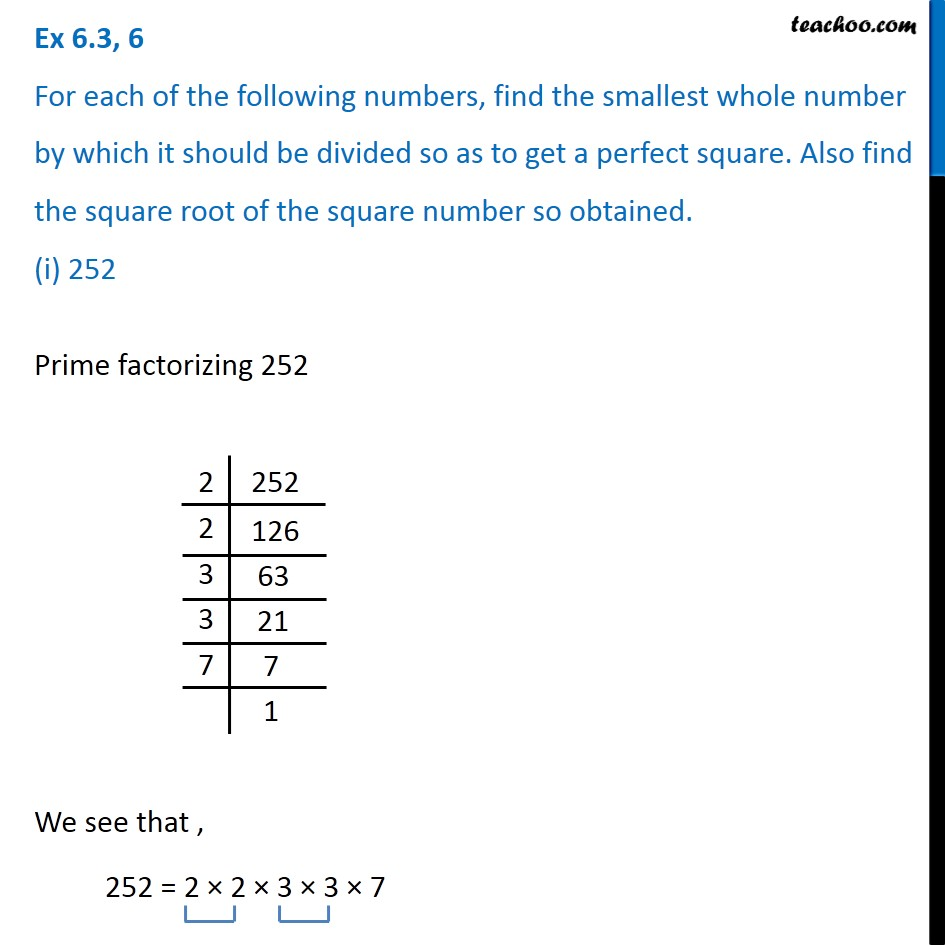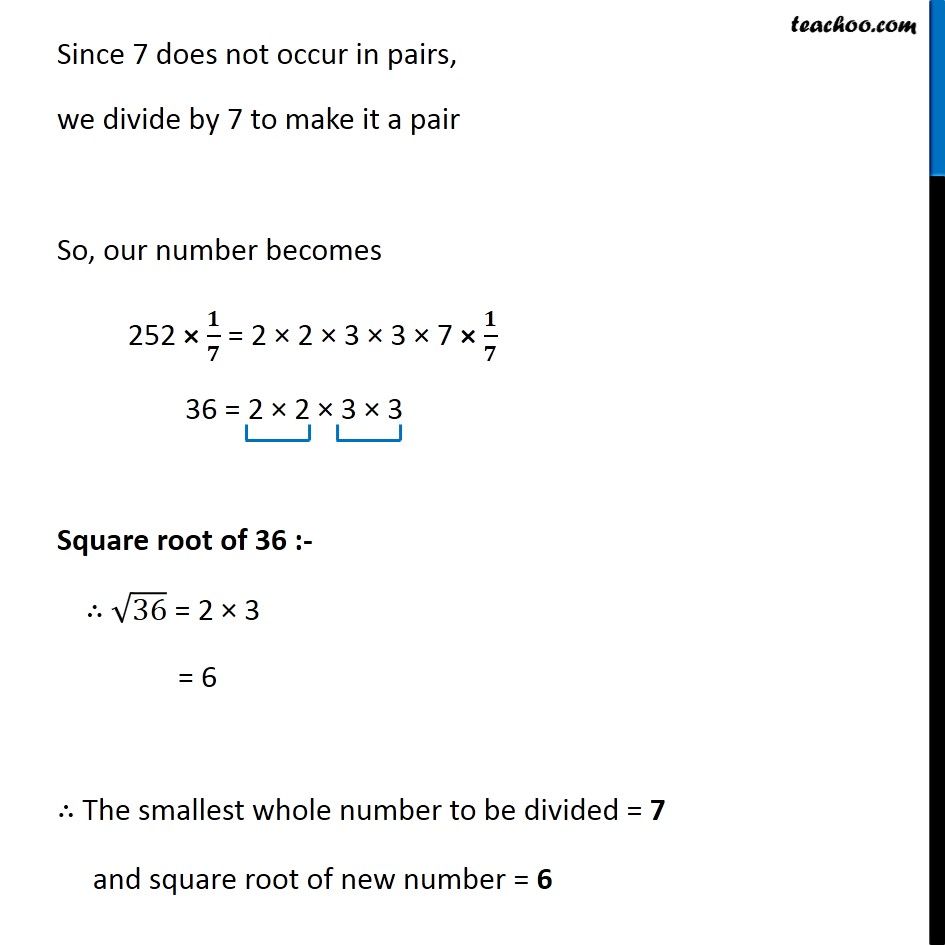1. Chapter 6 Class 8 Squares and Square Roots
2. Concept wise
3. Smallest number divided to get perfect square

Transcript

Ex 6.3, 6 For each of the following numbers, find the smallest whole number by which it should be divided so as to get a perfect square. Also find the square root of the square number so obtained. (i) 252Prime factorizing 252 We see that , 252 = 2 × 2 × 3 × 3 × 7 Since 7 does not occur in pairs, we divide by 7 to make it a pair So, our number becomes 252 × 𝟏/𝟕 = 2 × 2 × 3 × 3 × 7 × 𝟏/𝟕 36 = 2 × 2 × 3 × 3 Square root of 36 :- ∴ √36 = 2 × 3 = 6 ∴ The smallest whole number to be divided = 7 and square root of new number = 6